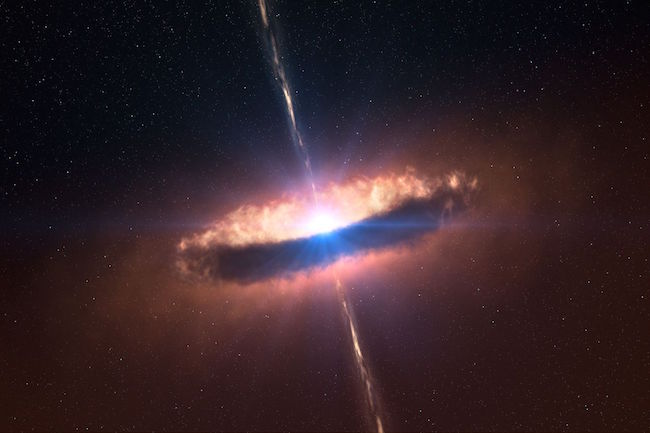# A star is bornA large, spherical, and very diffuse cloud of molecular hydrogen sits in empty space. The radius of the cloud is $r_0$ and the molecules are initially at rest. Let $t_0$ be the time it takes for this cloud to completely collapse, i.e. the time it takes for a molecule at $r_0$ to free-fall into the origin. Similarly, let $t_1$ be the time it takes for a cloud of radius $r_1=4r_0$ to collapse.

What is $\frac{t_1}{t_0}?$

Details and Assumptions:

• The entire cloud is collapsing in this process.
• Both clouds have the same mass.
×